# Molniya orbit

Molniya orbit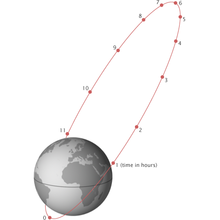Figure 1: The Molniya orbit. Usually the period from perigee + 2 hours to perigee + 10 hours is used to transmit to the northern hemisphere

Molniya orbit is a type of highly elliptical orbit with an inclination of 63.4 degrees, an argument of perigee of -90 degree and an orbital period of one half of a sidereal day. Molniya orbits are named after a series of Soviet/Russian Molniya (Russian: "Lightning") communications satellites which have been using this type of orbit since the mid 1960s.

A satellite in a highly eccentric orbit spends most of its time in the neighborhood of apogee which for a Molniya orbit is over the northern hemisphere, the sub-satellite point at apogee having a latitude of 63.4 degrees North. As the apogee altitude is as high as 40000 km it will therefore, for a considerable period around apogee, have an excellent visibility from the Northern hemisphere, from Russia but also from northern Europe, Greenland and Canada.

To get a continuous high elevation coverage of the northern hemisphere at least three Molniya spacecraft are needed.

The reason why the inclination should have the value 63.4 deg is that then the argument of perigee is not perturbed by the J2 term of the gravitational field of the Earth but stays -90 deg.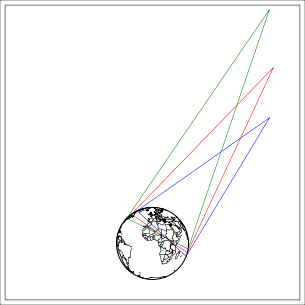Figure 2: Illumination zones (at least 10 deg elevation) from a Molniya orbit. At apogee the green illumination zone applies, 3 hours before or after apogee the red zone applies, 4 hours before or after apogee the blue zone applies. The plane of the figure is the longitudinal plane of apogee rotating with the Earth. In the eight hours period centered at the apogee passage the longitudinal plane is almost fixed, the longitude of the satellite varies with only +-2.7 deg. The views of the Earth from these 3 points are displayed in figures 3-8Figure 3: View of the Earth 4 hours before apogee from a Molniya orbit under the assumption that the longitude of the apogee is 90 deg E. The spacecraft is at an altitude of 24043 km over the point 92.65 deg E 47.04 deg N.Figure 4: View of the Earth from the apogee of a Molniya orbit under the assumption that the longitude of the apogee is 90 deg E. The spacecraft is at an altitude of 39867 km over the point 90 deg E 63.43 deg N.Figure 5: View of the Earth 4 hours after apogee from a Molniya orbit under the assumption that the longitude of the apogee is 90 deg E. The spacecraft is at an altitude of 24043 km over the point 87.35 deg E 47.04 deg N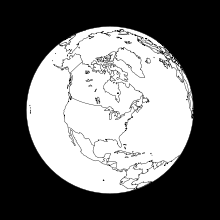Figure 6: View of the Earth 4 hours before apogee from a Molniya orbit under the assumption that the longitude of the apogee is 90 deg W. The spacecraft is at an altitude of 24043 km over the point 87.35 deg W 47.04 deg N.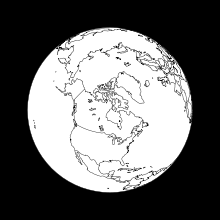Figure 7: View of the Earth from the apogee of a Molniya orbit under the assumption that the longitude of the apogee is 90 deg W. The spacecraft is at an altitude of 39867 km over the point 90 deg W 63.43 deg N.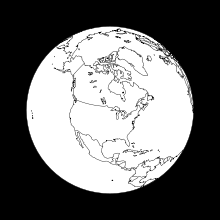Figure 8: View of the Earth 4 hours after apogee from a Molniya orbit under the assumption that the longitude of the apogee is 90 deg W. The spacecraft is at an altitude of 24043 km over the point 92.65 deg W 47.04 deg NFigure 9: A constellation of 3 Molniya spacecraft providing service for the Northern hemisphere. P is the orbital period. A green line corresponds to service for Asia and Europe with the visibility of figures 3-5. A red line corresponds to service for Northern America with the visibility of figures 6-8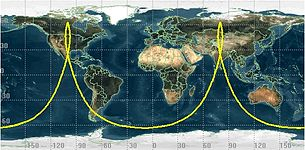Figure 10: Groundtrack of Molniya orbit. In the operational part of the orbit from apogee -3 hours to apogee +3 hours the satellite is north of 55.5 deg N (latitude of for example central Scotland, Moscow and southern part of Hudson Bay)

## Properties

Much of the area of the former Soviet Union, and Russia in particular, is located at high latitudes. To broadcast to these latitudes from a geostationary orbit would require considerable power due to the low elevation angles. A satellite in a Molniya orbit is better suited to communications in these regions because it looks directly down on them. In fact, in the period from apogee -3 hours to apogee +3 hours the sub-satellite point of the spacecraft is north of latitude 55.5 deg N and the elevation of the spacecraft is over 10 deg from all points north of latitude 54.1 deg N and over 5 deg from all points north of latitude 49.2 deg N.

An additional advantage is that considerably less launch energy is needed to bring a spacecraft into a Molniya orbit than into a geostationary orbit. Disadvantages are that as opposed to a spacecraft in a geostationary orbit the ground station needs a steerable antenna to track the spacecraft and that the spacecraft will pass the Van Allen belt 4 times per day.

It is necessary to have at least three spacecraft if permanent high elevation coverage is needed for a large area like the whole of Russia where some parts are as far south as 45 deg N. If three spacecraft are used each spacecraft is active for periods of 8 hours per orbit centered at apogee as illustrated in figure 9. As the Earth rotates half a revolution in 12 hours every second apogee will serve one half of the northern hemisphere and every second the other half. If for example the apogee longitudes are 90 deg E and 90 deg W this means that every second apogee will serve Europe and Asia (see figures 3 to 5) and every second Northern America (see figures 6 to 8). The orbits of the three spacecraft should then have the same apogee longitudes (for example 90 deg W and 90 deg E) but pass the apogee with 8 hours shift, i.e. the right ascensions of the ascending nodes should be separated with 120 deg. When one spacecraft has reached the point corresponding to figure 5 (or figure 8) 4 hours after apogee passage the next spacecraft having 4 hours left to reach apogee and having a right ascension of ascending node 120 deg larger then the previous spacecraft has the visibility displayed in figure 3 (or figure 6) and the switch-over can take place. Note that the two spacecraft at the time of switch-over only are separated with about 1500 km and that the ground stations therefore only have to move the antennas a few degrees to acquire the new spacecraft.

In general, the oblateness of the Earth perturbs the argument of perigee, so that even if the apogee started near the north pole, it would gradually move unless constantly corrected with "station keeping" thruster burns. To avoid this expenditure of fuel, the Molniya orbit uses an inclination of 63.4°, for which these perturbations are zero. That this is the case follows from equation (28) of the article Orbital perturbation analysis (spacecraft) as the factor$\left(\frac{5}{4}\ \sin^2 i\ -\ 1\right)$

then is zero.

The reason why the orbital period shall be half a sidereal day is that the geometry relative to the ground stations should repeat every 24 hours keeping the longitudes for the apogees passages. In fact, the precise ideal orbital period resulting in a ground track repeating every 24 hours is not precisely half a sidereal day but rather half a "nodal day"! The J2 term of the gravitational field of the Earth causes secular perturbations of both the right ascension of the ascending node$\Omega\,$ and the argument of perigee$\omega\,$, the formulas giving the change per orbital revolution (in radians) being$\Delta \Omega\ =\ -2\pi\ \frac{J_2}{\mu\ p^2}\ \frac{3}{2}\ \cos i$$\Delta \omega\ =\ \ -2\pi\ \frac {J_2}{\mu\ p^2}\ 3 \left(\frac{5}{4}\ \sin^2 i\ -\ 1\right)$

which are equations (24) and (28) of the article Orbital perturbation analysis (spacecraft)

For a Molniya orbit the inclination is selected such that$\Delta \omega\,$ as given by the formula above is zero but$\Delta \Omega\,$ as given by the other equation will be -0.0742 deg per orbit. The rotational period of the Earth relative the node, i.e. the "nodal day", will therefore be only 86129 seconds, 35 seconds less then the sidereal day which is 86164 seconds.

## Uses

The primary use of the Molniya orbit was for the communications satellite series of the same name. After two launch failures in 1964, the first successful satellite to use this orbit was Molniya 1-01 launched on April 23, 1965. The early Molniya-1 satellites were used for long-range military communications starting in 1968, but the satellites had a short lifespan and had to be constantly replaced. Its replacement, the Molniya-2, provided both military and civilian broadcasting, and was used to create the Orbita television network, spanning the Soviet Union. These were in turn replaced by the Molniya-3 design. There is some confusion in the existing sources about the naming, with some sources suggesting that all of the satellites on-orbit are of the Molniya-3 type, but referred to as Molniya-1 through -3 depending on their purpose.

The same orbits, with slight adjustments, were also used by some Soviet spy satellites, with the apogee point over the continental United States. Although geostationary orbits are useful for observing the continental United States, Soviet sensor technology sometimes required high-contrast observing angles which could only be achieved from higher latitudes. One such example is the US-KS early-warning satellite which watches for US missile launches, although improvements in these systems have since allowed them to move these to geostationary orbits.

Wikimedia Foundation. 2010.

Поможем написать реферат

### Look at other dictionaries:

• Molniya-1 No.2 — Major contractors OKB 1 Bus Molniya 1 Mission type Communications Launch date 4 June 1964 05:00 UTC Carrier rocket …   Wikipedia

• Molniya — (Russian молния for lightning) may refer to: The Molniya (satellite), the Soviet military communications satellite. Molniya orbit, distinctive orbit associated with the satellite. The Molniya (rocket), a four stage variation of the Soyuz launch… …   Wikipedia

• Orbit — This article is about orbits in celestial mechanics, due to gravity. For other uses, see Orbit (disambiguation). A satellite orbiting the Earth has a tangential velocity and an inward acceleration …   Wikipedia

• Molniya-M — For other uses, see Molniya (disambiguation). Molniya M (R 7 8K78M) Drawing of the Molniya M carrier rocket Function Medium carrier rocket Manufacturer TsSKB Progress Country of origin Russia/ …   Wikipedia

• Molniya (rocket) — For other uses, see Molniya (disambiguation). Molniya 8K78 was a modification of the well known R 7 Semyorka rocket and had four stages. This derivative of the original three stage Vostok rocket was especially designed to bring high flying… …   Wikipedia

• Orbit of the Moon — Not to be confused with Lunar orbit in the sense of a selenocentric orbit, that is, an orbit around the Moon The Moon completes its orbit around the Earth in approximately 27.3 days (a sidereal month). The Earth and Moon orbit about their… …   Wikipedia

• Orbit equation — In astrodynamics an orbit equation defines the path of orbiting body around central body relative to , without specifying position as a function of time. Under standard assumptions, a body moving under the influence of a force, directed to a… …   Wikipedia

• Orbit phasing — In astrodynamics orbit phasing is the adjustment of the time position of spacecraft along its orbit, usually described as adjusting the orbiting spacecraft s true anomaly. This is predominantly used in satellite positioning, especially if the… …   Wikipedia

• Molniya — Molnija, (russisch „Blitz“, in der englischen Schreibweise Molniya oder Molnyia, ist aus der altnordischen Sprache Mjölnir entlehnt) ist: ein russischer Uhrenhersteller Molnija (Uhrenhersteller) eine exzentrische Umlaufbahn: Molnija oder Highly… …   Deutsch Wikipedia

• Geocentric orbit — Earth orbit redirects here. For the motion of the Earth around the Sun, see Earth s orbit. Earth orbiter redirects here. For the shuttle simulator, see Earth Orbiter 1. The following words may have more than one definition or other non Earth… …   Wikipedia